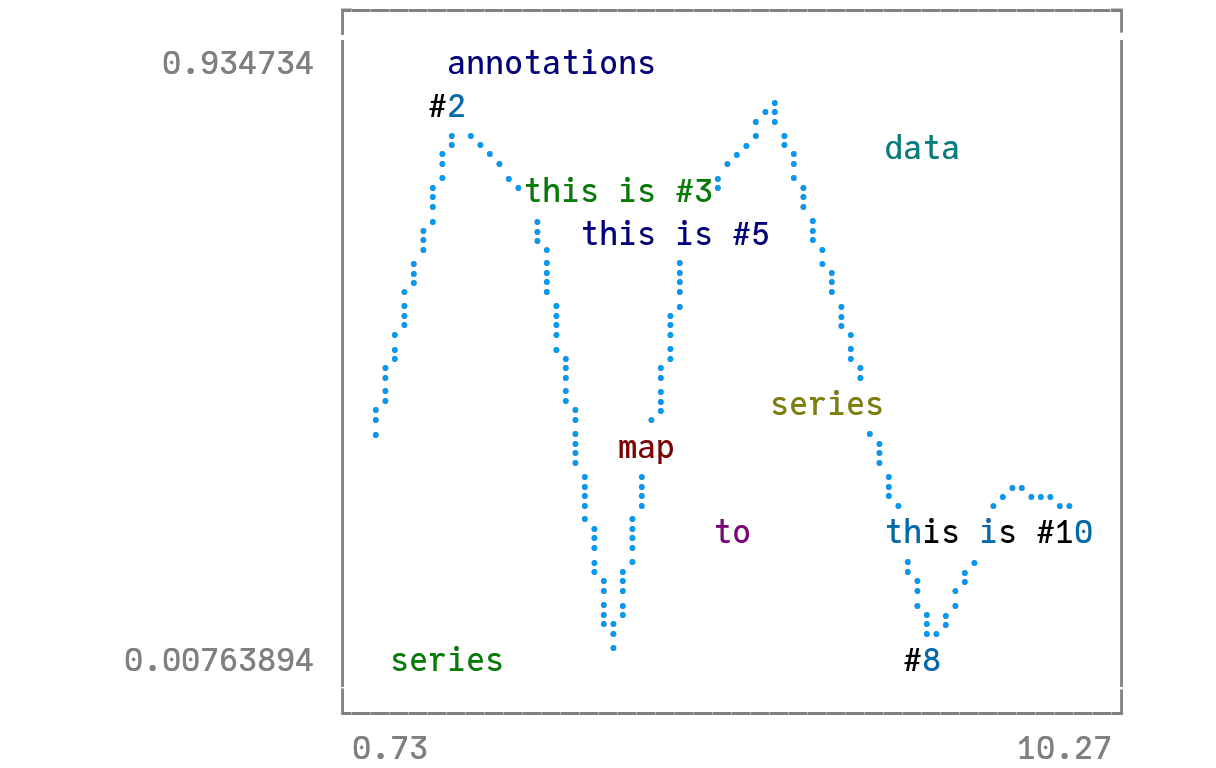# Annotationsusing Plots
unicodeplots()

The annotations keyword is used for text annotations in data-coordinates. Pass in a 3-tuple of vectors (x, y, text), or a vector of annotations, each of which is a tuple of x, y and text. You can position annotations using relative coordinates with the syntax ((px, py), text), where for example px=.25 positions the annotation at 25% of the subplot's axis width. text may be a simple String, or a PlotText object, which can be built with the method text(string, attrs...). This wraps font and color attributes and allows you to set text styling. text may also be a tuple (string, attrs...) of arguments which are passed to Plots.text.

annotate!(ann) is shorthand for plot!(; annotation=ann), and annotate!(x, y, txt) for plot!(; annotation=(x,y,txt)).

Series annotations are used for annotating individual data points. They require only the annotation; x/y values are computed. Series annotations require either plain Strings or PlotText objects.

y = rand(10)
plot(y, annotations = (3, y, Plots.text("this is #3", :left)), leg = false)
annotate!([(5, y, ("this is #5", 16, :red, :center)), (10, y, ("this is #10", :right, 20, "courier"))])
annotate!([2, 8], y[[2, 8]], ["#2", "#8"])
scatter!(range(2, stop = 8, length = 6), rand(6), marker = (50, 0.2, :orange), series_annotations = ["series", "annotations", "map", "to", "series", Plots.text("data", :green)])This page was generated using DemoCards.jl and Literate.jl.